Last time on vector napkin math, we recovered a dot product using one shared reference point. Let’s take it exactly one step further: two shared reference points. Shocking! Novel! Amazing! 🎉

Yes yes, but Obi Wan reminds us it’s our first step into a larger world… using N reference points 😱.

If you recall, last time, we knew vector `A`. We also knew its dot product to `u` and `v`: `u.A` and `v.A`. We hoped to recover `u.v`.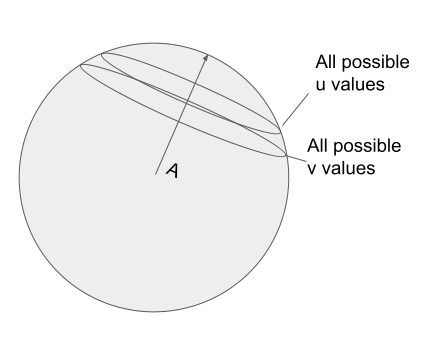We rotated `u` and `v` into `A`, so that the 0th dimension corresponds to `u.A` and `v.A`.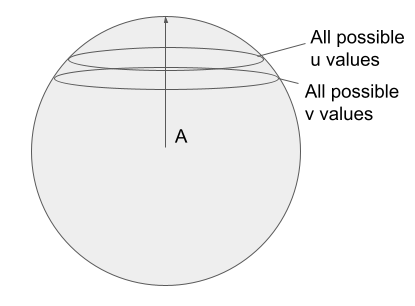0 1 n
u u.A ? ?
v v.A ? ?

And now the dot product:

`u.v = u.A*v.A + ?*? + ?*?...`

Since the dot product of the ??s at 1..n approaches 0, we know `u.v` must approach `u.A * u.v` as dimensionality increases.

Can we get a more accurate estimate? What if we don’t just have `A` but `A1...An` reference points, will that help?

Yes!

Let’s first look by just adding a second reference point, `A2`.

We can use our trick again, putting `u` and `v` into each reference points perspective.

Our original `A1`’s point of view:

0 1 n
u u.A1 ? ?
v v.A1 ? ?

Or, from `A2`’s vantage:

0 1 n
u u.A2 ?
v v.A2 ?

How do we combine these two perspectives to get an accurate estimate? Can we just do this?

0 1 n
u u.A1 u.A2 ?
v v.A1 v.A2 ?

And thus estimate `u.v=u.A1*v.A1 + u.A2*v.A2 + ...`

Can we do this?

We can only assume this when `A1.A2=0` (orthogonal). As `A1.A2` approaches 1 (parallel), simply summing `u.A1*v.A1 + u.A2*v.A2` double counts `A1`’s contribution to the dot product. `A2` just repeats what we know already from `A1`.

`A1` and `A2` being nearly parallel would be like two neighbors estimating a boat’s position. The first measures a boat’s distance as 4 km due east. Their neighbor measures the boat as 4.5KM Northeast. They can’t just sum their observations (as in `u.v=u.A1*v.A1 + u.A2*v.A2`) and assume the boat is 8.5 km away.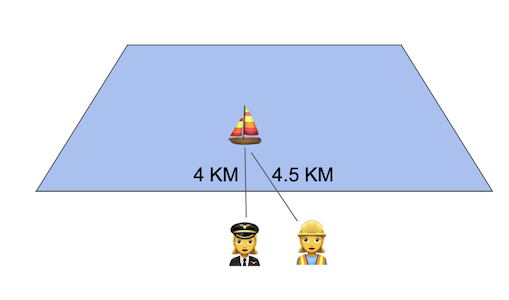BUT if two observers were observing the boat at close to 90 degrees apart, they could just add their observations. As they come from completely directions. One contributes information in the East-West dimension, the other in the North-South dimension.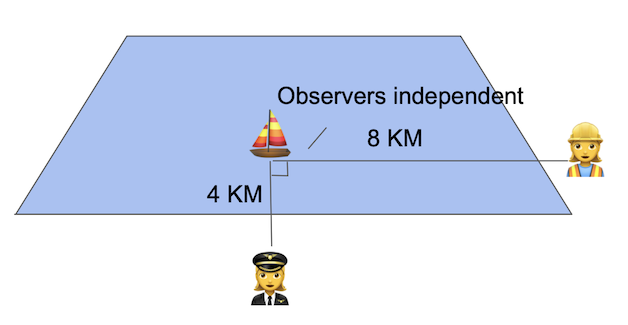Or in a more ‘vector’ formula:

East North
u 4.5km 8 km

In our triangulation of `u.v`, we want to plan for resilience. Reality will be somewhere in between these two situations. When `A1.A2`approaches 1 (parallel), we have miniscule amounts of new information. When `A1.A2` approaches 0 (orthogonal) we’ll want to incorporate 100% of the information.

We want to get the bits of A2’s observation that are orthogonal to A1. We want to account for the information not seen in the first observers vantage point of the distant boat. The dotted line below.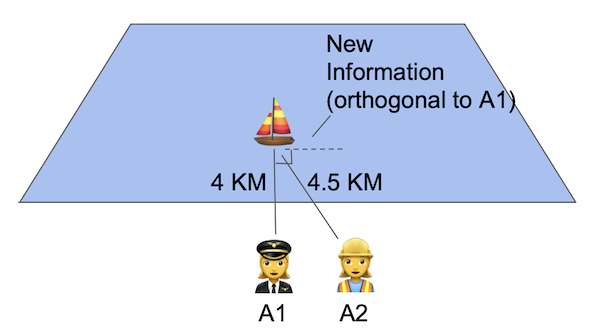Or the 1st dimension below.

0 1 n
u u.A1 Rotated(u.A2) ?
v v.A1 Rotated(v.A2) ?

Let’s do that!

Luckily, because we know `A1` and `A2` know the angle between A1 and A2: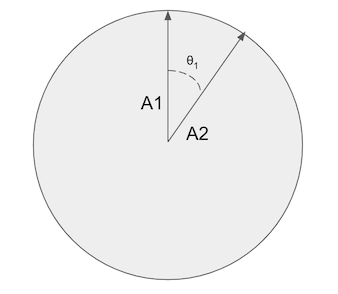You can imagine there’s some line that corresponds to axis 1, in the plane of A1/A2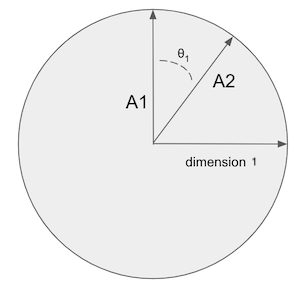And `u.A2` would correspond to some amount of magnitude along the A2 line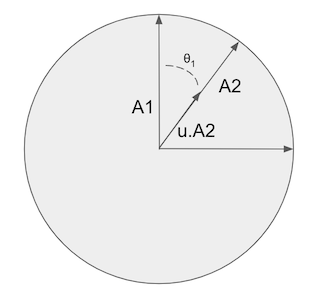We want to recover the projection of this line formed by `u.A2` onto the orthogonal line to `A1`. This extracts the independent information orthogonal to A1: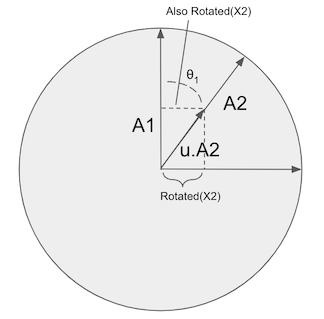Luckily, now this is just a basic trig problem

``````sin(θ1) = Opposite / Hypotenuse
Hypotenuse = u.A
Opposite = Rotated(u.A)

sin(θ1) = u.A / Rotated(X2)

Rotated(X2) = sin(θ1) * u.A
``````

And we now have these coordinates relative to A1:

0 1 n
u u.A1 u.A2 * sin(θ1) ?
v v.A1 v.A2 * sin(θ1) ?

Neat! Now we have a bit of new information for our dot product! Intuitively it corresponds to what we expect. If θ1 is closer to 90 degrees, we get more information - `sin(90°) == 1`. We’ll include all of `u.A2` in the dot product. Similarly for θ1 going to 0.

As the dot product of 2…n approaches 0, as dimensionality increases, we can expect the dot product to now approach `u.A1*v.A1 + u.A2 * sin(θ1) * v.A2 * sin(θ1)`

What if we want to add 3..N more reference points?

From the table above, you realize that `u.A1` and `u.A2` are a cosine, you can see this starts to resemble computing n-sphere coordinates from n-1 rotations, as described here. Wikipedia lists a handy set of equations:Adding a third reference point, `A3` should now be just a matter of continuing these equations:

0 1 2 n
u u.A1 u.A2 * sin(θ1) u.A3 * sin(θ1) * sin(θ2) ? ?
v v.A1 v.A2 * sin(θ1) u.A3 * sin(θ1) * sin(θ2) ? ?

Is that right?

Not quite. Imagine a case where θ1 is close to 0 (A1/A2 near parallel) but θ2 is close to 1 (near orthogonal, all new information!). If we followed this solution, dimension 2 above would go to 0. Yet that’s not what we expect. We have new information here given A3’s orthogonality.

What we really need is to find θ2 - the angle between the plane created by `A1,A2` and vector `A3`. Then repeat the same process we performed above.

0 1 2 n
u u.A1 u.A2 * sin(θ1) u.A3 * sin(θ2) ? ?
v v.A1 v.A2 * sin(θ1) u.A3 * sin(θ2) ? ?

The astute observer may see that the “rotated” dotted line in the pictures actually looks like an orthogonal projection which is your hint for extending to additional dimensions.

But this problem I’ll leave for another time :) Think you know the answer? Found a problem with my math? Feel free to get in touch. How do we find this?